/

### Accelerated Convergence Techniques

The term accelerated convergence appears in a number of different guises in mathematics and finance.

1.            In the context of numerical integration (also called quadrature), accelerated convergence can relate to approaches that aim to converge more rapidly to the correct answer as the number of evaluations,, of the underlying function increases.

Perhaps the most important example is what is more classically known as Simpson’s rule. The theory can be developed as follows. The simplest and perhaps most obvious way of evaluating an integral numerically is the so-called trapezoidal rule. Suppose we are trying to evaluate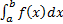and the abscissas (i.e. the points at which the function is evaluated) are equally spaced, say at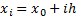for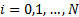(so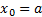and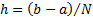), with the values of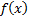there being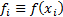. The trapezoidal rule involves approximating the integral by the following formula: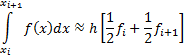The accuracy of this approximation can be expressed as follows: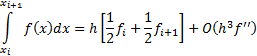By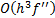we mean that the true answer differs from the estimate by an amount that is the product of some numerical coefficient times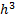times the value of the second derivative of the function somewhere in the interval of the integration (assuming that the function is sufficiently smooth and actually has a second derivative).

Repeated application of the above over each of the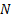parts of the overall integration results in the extended trapezoidal rule, namely: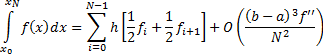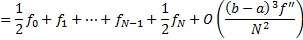Here we have written the error estimate in terms of the overall interval size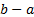andrather than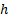as usuallywill be fixed andvaried to achieve a desired level of accuracy. This highlights that the accuracy of the trapezoidal rule improves by a factor of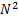asincreases (if the function being integrated is sufficiently smooth).

The trapezoidal rule is a two point formula (in the sense that each underlying interval involves just two points,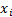and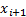) and is exact for polynomials up to and including degree 1, i.e.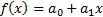(i.e. straight lines). We might therefore expect that there is a three point formula that is exact up to polynomials of degree two (i.e. quadratics). This is indeed the case, and it involves Simpson’s rule (here we use the convention that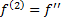,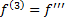,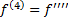etc.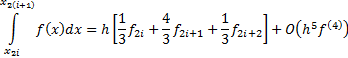Classically, a variety of other formulae were developed that fitted higher and higher order polynomials, e.g. Simpson’s 3/8 rule, Bode’s rule etc., but in practice the (extended) trapezoidal rule has some subtle but important facets which make it the starting point for many different numerical integration techniques, see e.g. Press et al. (2007). In particular, the error of the approximation which begins with a term of order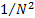is entirely even when expressed in powers of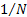.

Suppose, therefore, that we evaluate the trapezoidal rule withsteps (i.e.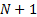abcissae) and come up with an answer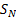. Suppose we repeat it with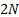steps (i.e. theabcissae previously used plusfurther ones equally spaced between them) and come up with an answer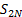. Then, if the function is sufficiently smooth, the leading error term in the second evaluation will be one-quarter of the size of the leading error in the first evaluation. We should therefore be able to test for convergence by repeatedly doubling the number of terms evaluated, and we should be able cancel out entirely the leading order error term using the combination: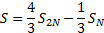The residual error term is of order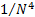, the same as Simpson’s rule; indeed it should not take long to realise that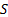is exactly the same as Simpson’s rule.

Various other refinements of this idea can be used to improve convergence further, e.g. Romberg integration.

2.            Alternatively, accelerated convergence can relate to other more general methods of improving the convergence properties of a series, i.e. where we approach the same end answer but with fewer evaluations of the series.

For example, suppose we have two convergent series, say: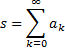and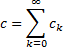with the property that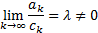Then Kummer’s transformation indicates that a series with a more rapid convergence to the same value is given by the following, see Abramowitz and Stegun (1972):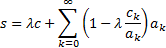This becomes particularly helpful ifis known already; useful cases include: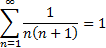and more generally: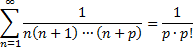Other examples of convergence improvement are given in e.g. Weisstein (2015).